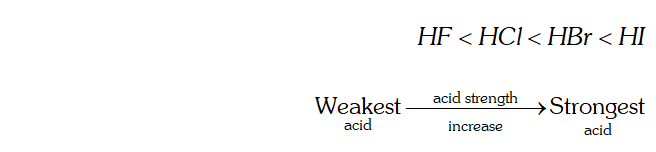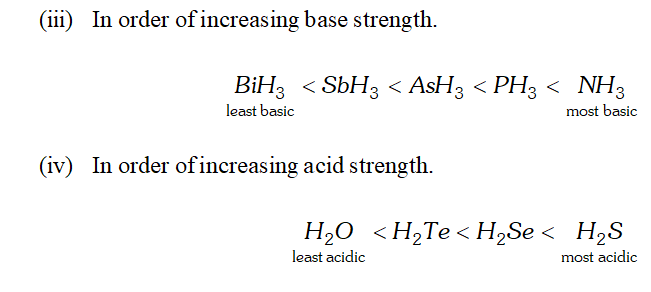Most Affordable JEE | NEET | 8,9,10 Preparation by Kota's Top IITian Doctor Faculties

# Noble Gases | Question Bank for Class 12 ChemistryQ. Why is $N_{2}$ less reactive at room temperature?
Ans. Due to the presence of a triple bond between the two nitrogen atoms, the bond dissociation energy of $N_{2}\left(941.4 \mathrm{kJ} \mathrm{mol}^{-1}\right)$ is very high. Therefore, $N_{2}$ is less reactive at room temperature.
Q. How does ammonia react with a solution of $C u^{2+}$ ion $?$
Ans. Ammonia forms a soluble complex with $C u^{2+}$ ion. $C u^{2+}+4 \ddot{N} H_{3} \rightarrow\left[C u\left(N H_{3}\right)_{4}\right]^{2+}$
Q. What happens when white phosphorus is heated with concentrated $\mathrm{NaOH}$ solution in an inert atmosphere of $\mathrm{CO}_{2} ?$
Ans. White phosphorus reacts with $\mathrm{NaOH}$ to form phosphine.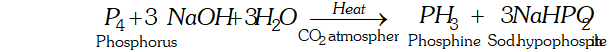Q. What is the basicity of $H_{3} P O_{4} ?$
Ans. - The structure of $H_{3} P O_{4}$ is tetrahedral. It has three $P-O H$ and one $P=O$ bond as shown below : since it has three ionizable $P-O H$ bonds, therefore $H_{3} P O_{4}$ is tribasic as shown below: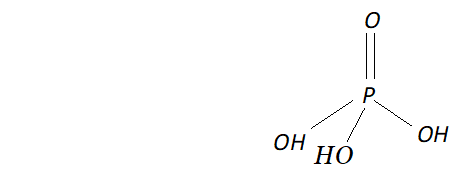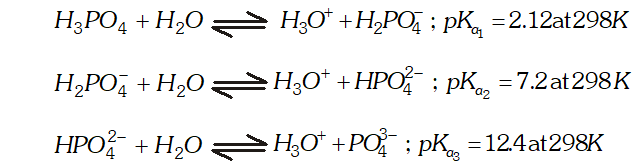since it is tribasic, it forms three series of salts with $N a O H$ viz, $\mathrm{NaH}_{2} \mathrm{PO}_{4}, \mathrm{Na}_{2} \mathrm{HPO}_{4}$ and $\mathrm{Na}_{3} \mathrm{PO}_{4}$
Q. Which of the following does not react with oxygen directly? $Z n, T i, P t, F e$
Ans. Platinum is a noble metal. The sum of its first four ionization enthalpies is very large. Therefore, it does not react with oxygen directly. In contrast $Z n, T i$ and $F e$ are active metals and hence directly react with oxygen to form their respective oxides.
Q. Sea is the greatest source of halogens. Comment.
Ans. Sea water contains chlorides, bromides and iodides of sodium, potassium, magnesium and calcium, but is mainly sodium chloride solution $(2.5 \%$ by mass). Dried up sea beds contain sodium chloride and carnallite, $K C l . M g C l_{2} .6 H_{2} O .$ Certain seaweeds contain upto $0.5 \%$ of iodine as sodium iodide and chile saltpetre $\left(N a N O_{3}\right)$ contain upto $0.2 \%$ of sodium iodate. Thus, sea is the greatest source of halogens.
Q. Give the reason for the bleaching action of $C l_{2}$
Ans. In presence of moisture or in aqueous solution, $C l_{2}$ liberates nascent oxygen.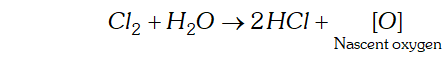This nascent oxygen brings about the oxidation of coloured substances present in vegetable and organic matter to colourless substances. Coloured substance $+O \rightarrow$ Colourless substance Thus, the bleaching action of is due to oxidation. Since the bleaching effect of is permanent, it is used to bleach cotton and textiles and wood pulp for manufacture of paper and rayon.
Q. Name two poisonous gases which can be prepared from chlorine gas.
Ans. poisonous gases which can be prepared from $C l_{2}$ are: (i) Phosgene $\quad$ (ii) $\quad$ Mustard gas. These are prepared as follows: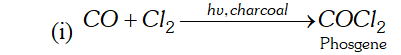(ii) is passed through boiling $S,$ when is formed. This when reacted with ethene gives mustard gas.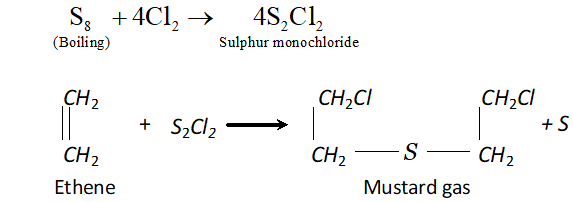Q. Why is $I C l$ more reactive than $I_{2} ?$
Ans. ICl is more reactive than $I_{2}$ because $I-C l$ bond is weaker than $I-I$ bond. Consequently, $I C l$ breaks easily to form halogen atoms which readily bring about the reastions.
Q. Why is helium used in diving apparatus?
Ans. - Because of its low solubility (as compared to $\left.N_{2}\right)$ in blood, a mixture of oxygen and helium is used in diving apparatus used by deep sea divers.
Q. Balance the following equation: $X e F_{\delta}+H_{2} O \rightarrow X e O_{2} F_{2}+H F$
Ans. $\mathrm{XeF}_{6}+3 \mathrm{H}_{2} \mathrm{O} \rightarrow \mathrm{XeO}_{3}+6 \mathrm{HF}$
Q. Why has it been difficult to study the chemistry of radon?
Ans. - Because radon is a radioactive element with a short half life of 3.82 days. This makes the study of chemistry of radon difficult.
Q. Write the reaction of thermal decomposition of sodium azide.
Ans. Sodium azide decomposes to form sodium metal and $N_{2}$ gas.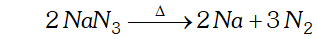Q. Why does $N H_{3}$ act as a Lewis base?
Ans. N is $N H_{3}$ has one lone pair of electrons which is available for donation. Therefore, it donates an electron pair to a proton to form $N H_{4}^{+}$ ion and hence it acts as a Lewis base.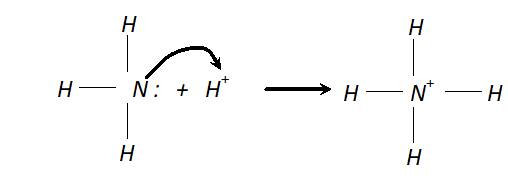Q. Why does $N O_{2}$ dimerise?
Ans. $\mathrm{NO}_{2}$ is an odd electron $(7+2 \times 8=23)$ molecule. In the valence shell, $N$ has seven electrons and hence is unstable. To become stable by having even ( 8) number of electrons in the valence shell, it undergoes dimerization to form $N_{2} O_{4}$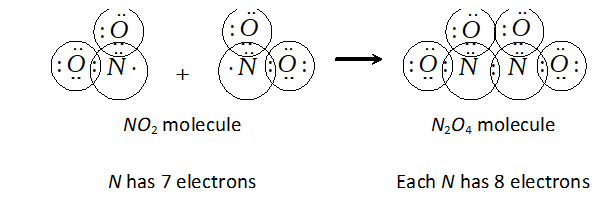Q. In what way it can be proved that $P H_{3}$ is basic in nature?
Ans. $P H_{3}$ reacts with acids like $H I$ to form $P H_{4} I .$ This shows that is basic in nature.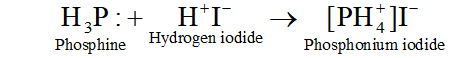Due to the presence of a lone pair of electrons on $P H_{3}$ is acting as a Lewis base in the above reaction.
Q. Why does $P C l_{3}$ fume in moisture?
Ans. In presence of moisture $\left(H_{2} \mathrm{O}\right), P C l_{3}$ undergoes hydrolysis giving fumes of $H C l$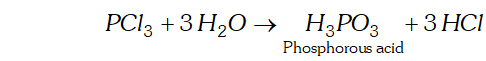Q. $H_{2} S$ is less acidic then $H_{2} T e$ why?
Ans. - As the size of the element increases down the group, $E-H$ bond distance increases and hence $E-H$ bond dissociation energy decreases. In other words, $H-S$ bond dissociation energy is higher than that of $H-T e$ bond dissociation energy and hence $H-S$ bond breaks less easily than $H-$ Te bond. Therefore, $H_{2} S$ is a weaker acid than $\mathrm{H}_{2} \mathrm{Te}$
Q. When $H C /$ reacts with finely powdered iron, it forms ferrous chloride and not ferric chloride. Why?
Ans. Fe reacts with $\mathrm{HCl}$ to form $\mathrm{FeCl}_{2}$ and $\mathrm{H}_{2}$ $$F e+2 H C l \rightarrow F e C l_{2}+H_{2}$$ thus liberated prevents the oxidation of to
Q. Unlike phosphorus, nitrogen shows little tendency for catenation?
Ans. Due to smaller size, the lone pair of electrons on the $N$ -atoms repel the bond pair of $N-N$ bond. In contrast, because of comparatively larger size of $P,$ the lone pair of electrons on $P$ atoms do not repel the bond pair of the $P-P$ bond to the same extent. As a result, $N_{-}$ $N$ single bond is weaker than $P-P$ single bond. Consequently nitrogen shows little tendency for catenation while $P$ has a distinct tendency for catenation.
Q. Give the disproportionation reaction of $H_{3} P O_{3}$
Ans. Phosphorus acid $\left(H_{3} P O_{3}\right)$ when heated at $473 K$ disproportionate to form orthophosphoric acid and phosphine.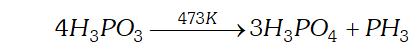Q. Explain why inspite of nearly the same electronegativity, oxygen forms hydrogen bonding while chlorine does not.
Ans. - Oxygen atom can form $H$ bonds whereas chlorine does not. The tendency for $H$ bonding depends upon (i) Small size and (ii) High electronegativity values Although the electronegativity of $O$ and $C l$ is nearly same yet chlorine does not form $H$ bond due to its larger size (99 pm) as compared to oxygen $(66 \mathrm{pm})$
Q. How can you prepare $\mathrm{Cl}_{2}$ from $\mathrm{HCl}$ and $\mathrm{HCl}$ from $\mathrm{Cl}_{2} ?$ Write reactions only.
Ans. (i) Chlorine can be obtained from $H C l$ by heating with oxidising agents like $M n O_{2}, K M n O_{4}, K_{2} C r_{2} O_{7}, O_{3}$ etc.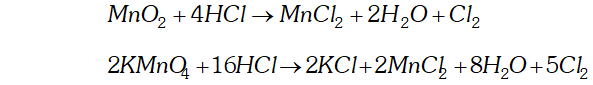(ii) $\quad H C l$ can be obtained from chlorine by burning it in excess of hydrogen $H_{2}+C l_{2} \rightarrow 2 H C l$
Q. With what neutral molecule is $\mathrm{ClO}^{-}$ isoelectronic ? Is that molecule a Lewis Base?
Ans. $\mathrm{ClO}^{-}$ species has 26 electrons $(17(\mathrm{Cl})+8(\mathrm{O})+1 \bar{e}$ (charge). The meutral molecule which is isoelectronic with it is $C I F(17+9=26 \bar{e})$ ClF can act as a lewis base due to presence of lone pair of electrons.
Q. Which one of the following does not exist?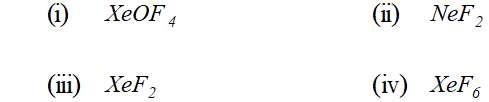Ans. (ii) $\quad$ NeF $_{2}$ cannot exist because Neon $(z=10)$ do not contain vacant $d$ -orbitals in its valence shell and hence cannot extend its valency.
Q. Why are pentahalides more covalent than trihalides ?
Ans. The elements of group 15 have five electrons (two in the $s$ -and three in the $p \text { -orbitals })$ in their respective valence shells. since it is difficult to lose all the three electrons to form $E^{3+}$ or even more difficult to lose all the five valence electrons (two $s$ -and three $p-$ ) to $E^{5+}$ ions, therefore, higher elements have no tendency to form ionic compounds. Instead they form covalent compounds by sharing of electrons. since elements in the $+5$ oxidation state have less tendency to lose electrons than in the $+3$ oxidation state, therefore, elements in the $+5$ oxidation state have more tendency to share electronts in the the $+3$ oxidation state. Thus, elements in the $+5$ oxidation state are $_{j}$ more covalent than in the $+3$ oxidation state. In other words, pentahalides are more covalent than trihalides.
Q. Why is $B i H_{3}$ the strongest reducing agent amongst all the hydrides of Group 15 elements?
Ans. As we move down the group, the size of the element increases and, therefore, the length of the $B-H$ bond increases and its strength decreases. In other words, as we move down the group, the $B-H$ bond can break more easily to evolve $H_{2}$ gas which acts as the reducing agent. Thus, $B i-H$ bonds is the weakest amongst the hydrides of elements of group 15 and hence $B i H_{3}$ is the strongest reducing agent.
Q. Mention the conditions to maximise the yield of ammonia.
Ans. Ammonia is prepared by the Haber's process.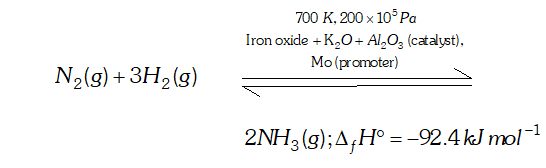In accordance with Le Chatelier's principle, to maximize the yield, a high pressure of $200 \times 10^{5} \mathrm{Pa}$ is used. To increase the rate of the reaction, a temperature of around $700 K$ is used and iron oxide mixed with some $K_{2} O$ and $A l_{2} O_{3}$ is used as a catalyst. Sometimes, Mo is also used as a promoter to increase the efficiency of the Fe catalyst.
Q. What is the covalency of nitrogen in $N_{2} O_{5} ?$
Ans. Covalency depends upon the number of shared pairs of electrons. Now in $N_{2} O_{5},$ each nitrogen atom has four shared paired of electrons as shown below: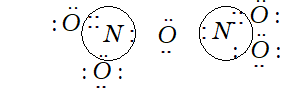Therefore, the covalency of $N$ in $N_{2} O_{5}$ is 4
Q. Bond angle in $P H_{4}^{+}$ is higher than in $P H_{3} .$ Why?
Ans. $P$ in $P H_{3}$ is $s p^{3}$ -hybridized. It has three bond pairs and one lone pair around $P .$ Due to greater lone pair-bond pair repulsion than bond pair-bond pair repulsion, the tetrahedral angle decreases from $109^{\circ}-28^{\prime}$ to $93.6^{\circ} .$ As a result, is pyramidal. However, when it reacts with a proton, it forms which has four bond pairs and no lone pair. Due to the absence of lone pair-bond pair repulsion and presence of four identical bond pair-bond pair interactions $\mathrm{PH}_{4}^{+}$ assumes tetrahedral geometry with a bond angle of $109^{\circ}-28^{\prime} .$ This explains why the bond angle in $\mathrm{PH}_{4}^{+}$ is higher than in $\mathrm{PH}_{3}$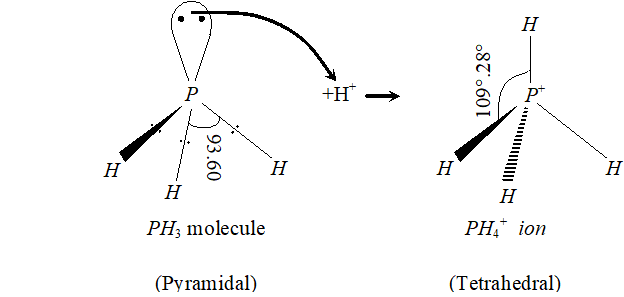Q. What happens when $P C l_{5}$ is heated?
Ans. $-P C l_{5}$ has three equatorial $(202 \text { pm long) and two axial }(240\text { pm }$ long) bond. since axial bonds are weaker than equatorial bonds, therefore, when $\mathrm{PCl}_{5}$ is heated, the less stable axial bonds break to form $\mathrm{PCl}_{3}$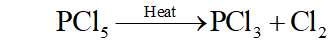Q. Write a balanced equation for the hydrolytic reaction of $P C l_{5}$ with heavy water.
Ans. It reacts with heavy water to form phosphorus oxychloride $\left(P O C l_{3}\right)$ and deuterium chloride $(D C l)$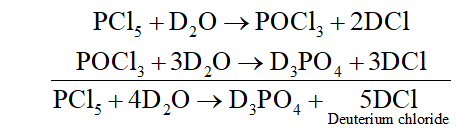Q. What happens when $H_{3} P O_{3}$ is heated?
Ans. The oxidation state of $P$ in $H_{3} P O_{3}$ is $+3 .$ since this value is intermediate between the highest $(+5)$ and lowest $(-3)$ oxidation states of $P,$ therefore, when is heated, it undergoes disproportionation to form and with oxidation states of $-3$ and $+5$ respectively.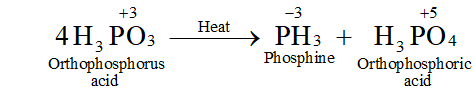Q. List the important sources of sulphur.
Ans. Sulphur mainly occurs in the earth's crust in the combined state primarily in form of sulphates and sulphides. Sulphates : Gypsum, $\quad \mathrm{CaSO}_{4} .2 \mathrm{H}_{2} \mathrm{O} \quad ;$ epsom salt $M g S O_{4} \cdot 7 H_{2} O ;$ baryte, $B a S O_{4},$ etc. Sulphides : Galena, $P b S ;$ zinc blende, $Z n S$; copper pyrites, CuFeS_{2} Traces of sulphur occur as $H_{2} S$ and in organic materials such as eggs, proteins, garlic, onion, mustard, hair and wool.
Q. Write the order of thermal stability of the hydrides of group 16 elements.
Ans. - As the size of the element increases down the group, the $E-H$ bond dissociation energy decreases and hence $E-H$ bond breaks more easily. Thus, the thermal stability of the hydrides of group 16 elements decreases down the group, $H_{2} O>H_{2} S>H_{2} S e>H_{2} T e>H_{2} P_{O}$
Q. Why is $H_{2} O$ liquid and $H_{2} S$ is a gas?
Ans. Due to greater electronegativity of $O$ than $S, H_{2} O$ under goes extensive intermolecular $H$ -bonding. As a result, exists as an associated molecule in which each $O$ is tetrahedrally surrounded by four water molecules. Quite a larger amount of energy is required to break these molecules. Therefore, is a liquid at room temperature.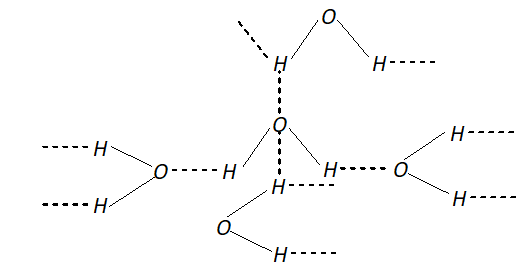Q. Complete the following reactions: (i) $\quad C_{2} H_{4}+O_{2} \rightarrow$ (ii) $4 A l+3 O_{2} \rightarrow$
Ans. (i) $C_{2} H_{4}$ undergoes combustion to form $C O_{2} \& H_{2} O$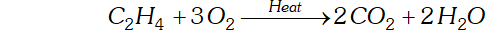(ii) $A l$ combines with $O_{2}$ to form alumina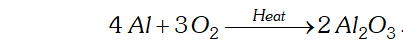Q. Why does ozone act as a powerful oxidising agent ?
Ans. $\mathrm{O}_{3}$ is an endothermic compound. On heating, it readily decomposes to give dioxygen and nascent oxygen.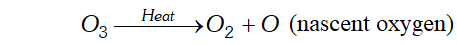since nascent oxygen is very reactive, therefore, $O_{3}$ acts as a powerful oxidising agent.
Q. How is $O_{3}$ estimated quantitatively?
Ans. When $O_{3}$ is treated with excess of $K I$ solution buffered with berate buffer (pH 9.2), I $_{2}$ is liberated quantitatively. $2 I^{-}(a q)+H_{2} O(l)+O_{3}(g) \rightarrow 2 O H^{-}(a q)+I_{2}(s)+O_{2}(g)$ The thus liberated is titrated against a standard solution of sodium thiosulphate using starch as an indicator.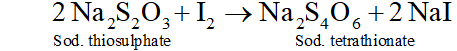Q. What happens when sulphur dioxide is passed into aqueous solution of $F e(\mathrm{III})$ salt $?$
Ans. $\mathrm{SO}_{2}$ acts as areducing agent and hence reduces an aqueous solution -of $F e(\text { III ) salt to } F e(I I)$ salt.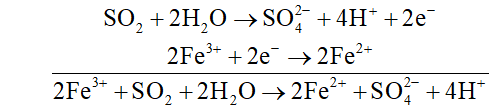Q. Comment upon the nature of two $S-O$ bonds formed in $S O_{2}$ molecule? Are the two $S-O$ bonds in this molecule equal?
Ans. In $S O_{2}, S$ is $s p^{2}$ -hybridized. Two of the three -orbitals form two $\sigma$ -bonds while the third contains the lone pair of electrons. $S$ is now left with one half-filled $p$ -orbital and one half-filled $d$ -orbitals. These form one and one double bond with oxygen atom. Thus, has bent structure with $O S O$ bond angle of $119.5^{\circ} .$ Due to resonance, the two bonds are equal $(143 \mathrm{pm})$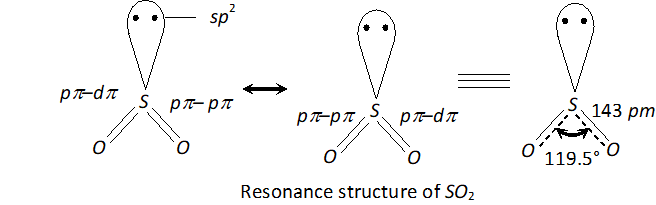Q. How is the presence of $S O_{2}$ detected?
Ans. $\mathrm{SO}_{2}$ is a pungent smelling gas. It can be detected by the following two tests : (i) turns the pink violet colour of $\mathrm{KMnO}_{4}$ to colourless due to reduction of $\mathrm{MnO}_{4}^{-}$ to $\mathrm{Mn}^{2+}$ ions.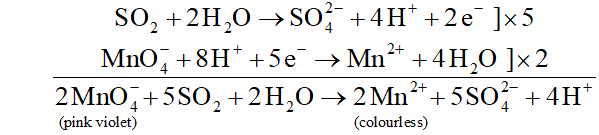(ii) $\mathrm{SO}_{2}$ turns acidified $\mathrm{K}_{2} \mathrm{Cr}_{2} \mathrm{O}_{7}$ green due to reduction of $\mathrm{Cr}_{2} \mathrm{O}_{7}^{2-}$ to $\mathrm{Cr}^{3+}$ ions.
Q. Mention three areas in which $H_{z} S O_{4}$ plays an important role.
Ans. (i) $\quad H_{2} \mathrm{SO}_{4}$ is used in the manufacture of fertilizers such as ammonium sulphate, calcium super phosphate. (ii) It is used as an electrolyte in storage batteries. (iii) It is used in petroleum refining, detergent industry and in the manufacture of paints, pigments and dyes.
Q. Write the conditions to maximise the yield of $H_{2} S O_{4}$ by contact process.
Ans. The key step in the production of $H_{2} S O_{4}$ is the oxidation of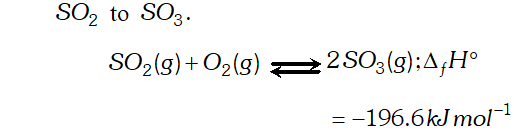The reaction is exothermic, reversible and the forward reaction proceeds with decrease in volume. Therefore, in accordance with Le chatelier's principle, to maximize the yield of $S O_{3}$ and hence of $H_{2} S O_{4},$ a low temperature $(720 \mathrm{K}$ to have optimum rate), a high pressure $( \left2 \text { bar) and } V_{2} O_{5}$ is used as a catalyst (to increase \right. the rate at $720 \mathrm{K}$ ).
Q. Why is $K_{a_{2}}$ less than $K_{a_{I}}$ for $H_{2} S O_{4}$ in water?
Ans. $H_{2} S O_{4}$ is a dibasic acid, it ionizes in two stages and hence has two dissociation constant.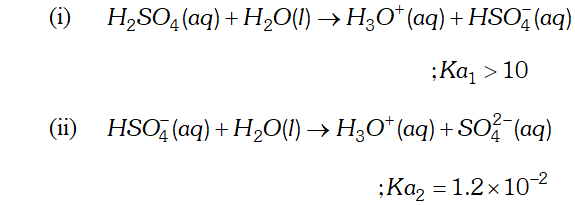$K_{a_{2}}$ is less than $K_{a_{1}}$ because the negatively charged $H S O_{4}^{-}$ ion has much less tendency to donate a proton to $H_{2} O$ as compared to neutral to donate a proton to water.
Q. Considering the parameters such as bond dissociation enthalpy, electron gain enthalpy and hydration energy, compare the oxidising power of $F_{2}$ and $C l_{2}$
Ans. The electrode potential of $F_{2}(+2.87 V)$ is much higher than that of $\mathrm{Cl}_{2}(+1.36 \mathrm{V}),$ therefore, $\mathrm{F}_{2}$ is a much stronger oxidising agent than $C l_{2} .$ Now, electrode potential depends upon three factors: (i) Bond dissociation energy, (ii) Electron gain enthalpy and (iii) Hydration energy. Although electron gain enthalpy of fluorine is less negative $\left(-333 \mathrm{kJ} \mathrm{mol}^{-1}\right)$ than that of chlorine $\left(-349 \mathrm{kJ} \mathrm{mol}^{-1}\right),$ the bond dissociation energy of $\mathrm{F}-\mathrm{F}$ bond is much lower $\left(158.8 \mathrm{kJ} \mathrm{mol}^{-1}\right)$ than that of $\mathrm{Cl}-\mathrm{Cl}$ bond $\left(242.6 \mathrm{kJ} \mathrm{mol}^{-}\right)$ and hydration energy of $\mathrm{F}^{-} \mathrm{ion}\left(515 \mathrm{kJ} \mathrm{mol}^{-1}\right)$ is much higher than that of $C l^{-}$ ion $\left(381 k J m o l^{-1}\right) .$ The later two factors more than compensate the less negative electron gain enthalpy of $F_{2} .$ As a result, electrode potential of is higher than that of chlorine and hence is a much stronger oxidising agent than
Q. Give two examples to show the anomalous behaviour of fluorine.
Ans. The anomalous behaviour of fluorine is due to its (i) Small size, (ii) Highest electronegativity, (iii) Low $F-F$ bond dissociation enthalpy and (iv) Non-availability of $d$ -orbitals in its valence shell. The two examples are: 1. Due to non-availability of $d$ -orbitals in its valence shell, fluorine cannot expand its octet, therefore, fluorine shows only an oxidation state of $-1$ while all other halogens due to the presence of $d$ -orbitals, show positive oxidation states of $+1,+3,+5$ and $+7$ besides oxidation state of $-1$ 2. Due to its small size, the three lone pair of electrons on each $F$ atom in $F-F$ molecule, repel the bond pair. As a result, $F-F$ bond dissociation energy is lower than that of $C l-C l$ bond.
Q. Though nitrogen exhibits $+5$ oxidation state, it does not form pentahalide, Give reason or Nitrogen does not form pentahalide. Explain.
Ans. The electronic configuration of nitrogen is $1 s^{2} 2 s^{2} 2 p_{x}^{1} 2 p_{y}^{1} 2 p_{z}^{1} .$ It has three half-filled $p$ -orbitals and hence can form a trihalide. To make a pentahalide, we need five half filled orbitals. Since nitrogen with $n=2$ can have only $s$ -and $p$ -orbitals and no $d$ -orbitals, it cannot expand its valence shell to show a covalency of $5 .$ At the same time, $N$ has a vacant $3 s$ orbital. If one of the $2 s$ -electron gets promoted to $3 s$ orbital, we can still have five half-filled orbitals to form $\mathrm{NCl}_{5}$. since energy required to promote one 2s electron to 3s orbital is more than the energy released during the formation of two additional bonds, therefore, such on excitation is thermodynamically not feasible. That is why nitrogen does not form a pentahalide.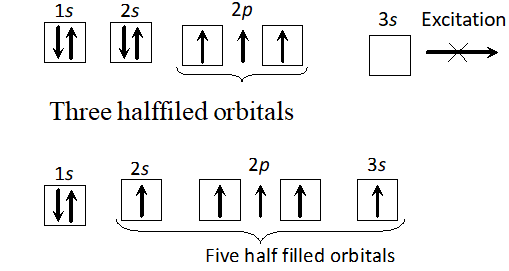Q. $P H_{3}$ has lower boiling point than $N H_{3} .$ Why?
Ans. The electronegativity of $N(3.0)$ is much higher than that of $P(2.1)$ Therefore, $N H_{3}$ undergoes extensive intermolecular $H$ -bonding and hence it exists as an associated molecule. To break these $H-$ bonds, a large amount of energy is needed. On the other hand, $P H_{3}$ does not undergo $H$ -bonding and thus exists as discrete molecules. Therefore, the boiling point of is much lower than that of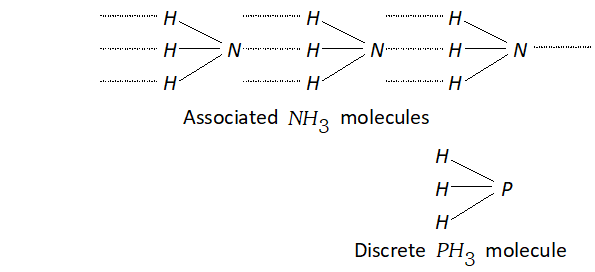Q. Are all the five bonds in $P C l_{5}$ equivalent? Justify.
Ans. $P C l_{5}$ has trigonal bipyramid structure. It has three equatorial bonds inclined at angle of $120^{\circ}$ and two axial bonds inclined at angle of $90^{\circ}$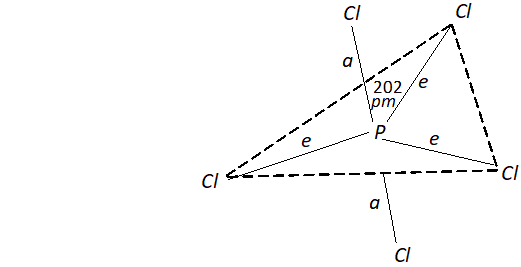As a result, axial bond pairs suffer greater repulsion than equatorial bond pairs. Consequently, axial bonds are longer $(240 p m)$ as compared to equatorial bonds $(202 p m) .$ Thus, all the five $P-C l$ bonds in $P C l_{5}$ are not equivalent.
Q. How do you account for the reducing behaviour of $\mathrm{H}_{3} \mathrm{PO}_{2} ?$
Ans. The structure of $H_{3} P O_{2}$ has two $P-H$ bonds. Due to the presence of these $P-H$ bonds $\mathrm{H}_{3} \mathrm{PO}_{2},$ acts as a strong reducing agent. For example, it reduces $\mathrm{AgNO}_{3}$ to $A g$ and arene diazonium salts to arenes.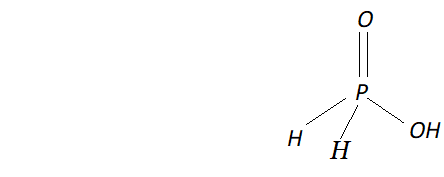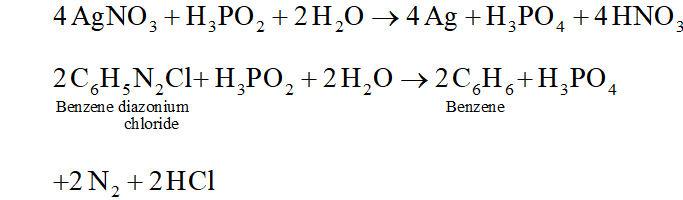Q. Elements of group 16 generally show lower value of first ionization enthalpy compared to the corresponding periods of group $15 .$ Why?
Ans. This is because of the following two reasons: (i) Due to extra stability of the exactly half-filled $p$ -orbitals electronic configuration of group 15 elements, they require larger amount of energy to remove an electron as compared to group 16 elements. (ii) After removal of an electron, the group 16 elements yield species which have more stable exactly half-filled $p$ -orbitals electronic configuration while this is not so in case of group 15 elements.
Q. Which form of sulphur shows paramagnetic behaviour? Or sulphur in the vapour state exhibits paramagnetism.
Ans. In the vapour state $(-1000 \mathrm{K}),$ sulphur partly exists as $S_{2}$ molecule which has two unpaired electrons in the antibonding $\pi^{*}$ -molecular orbitals like $O_{2}$ and hence, $\mathrm{S}_{2}$ exhibits paramagnetism.
Q. What happens when : (i) Concentrated $H_{2} S O_{4}$ is added to calcium fluoride: (ii) $\quad S O_{3}$ is passed through water?
Ans. (i) $\quad H_{2} \mathrm{SO}_{4}$ being less volatile displaces more volatile $H F$ from $C a F_{2}$ $\mathrm{CaF}_{2}+\mathrm{H}_{2} \mathrm{SO}_{4} \rightarrow \mathrm{CaSO}_{4}+2 \mathrm{HF}$ (ii) $\mathrm{SO}_{3}$ dissolves in water to form $\mathrm{H}_{2} \mathrm{SO}_{4}$ $$\mathrm{SO}_{3}+\mathrm{H}_{2} \mathrm{O} \rightarrow \mathrm{H}_{2} \mathrm{SO}_{4}$$
Q. Halogens have maximum negative electron gain enthalpy. Explain why?
Ans. Halogens have the smallest size (except noble gases) in their respective periods and, therefore, have high effective nuclear charge. As a result, they readily accept one electron to acquire the stable electronic configuration of the nearest noble gas. In other words, large amount of energy is released when a halogen atom accepts an electron to form the corresponding halide ion and thus halogens have maximum negative electron gain enthalpy.
Q. Although electron gain enthalpy of fluorine is less negative as compared to chlorine, fluorine is a stronger oxidising agent than chlorine. Why?
Ans. - The oxidising capability of a substance can be determined by its electrode potential. Higher the electrode potential, stronger is the oxidising agent. The electrode potential, in turn, depends of three factors : (i) Bond dissociation enthalpy (ii) Electron gain enthalpy (iii) Hydration energy Although electron gain enthalpy of fluorine is less negative than that of chlorine but (i) Enthalpy of dissociation of $F-F$ bond is lower than that of $C l-C l$ bond (ii) Hydration energy of $F^{-}$ ion is higher than that of $C l^{-}$ ion. Because of these two reasons, electrode potential of $F_{2}(+2.87 \mathrm{V})$ is muchhigher than that of $C l_{2}(+1.36 V)$ and hence $F_{2}$ is astronger oxidising agent than $C l_{2}$
Q. Fluorine exhibits only $-1$ oxidation state whereas other halogens exhibit $+1,+3,+5$ and $+7$ oxidation states. Explain.
Ans. Fluorine, like other halogens has seven electrons in the valence shell. since it is the most electronegative element, therefore, it readily accepts one electron to acquire the stable electronic configuration of the nearest noble gas. Therefore, like other halogens, it also exhibits an oxidation state of - 1 Further, since fluorine is the most electronegative element and does not have $d$ -orbitals in its valence shell, therefore, it cannot expand its octet and hence does not show positive oxidation states. On the other hand, other halogens have $d$ -orbitals and, therefore, ¡can expand their octets and thus show positive oxidation states of $+1,+3,+5$ and $+7$ in addition to oxidation state of $-1$ can expand their octets and thus show positive oxidation states of $+1,+3,+5$ and $+7$ in addition to oxidation state of $-1$
Q. Write the balanced chemical equation for the reaction of $C l_{2}$ with hot and concentrated $\mathrm{NaOH}$ ? Is this reaction a disproportionation reaction ? Justify.
Ans. This is an example of a disproportionation reaction because the oxidation state of chlorine decreases from zero in $C l_{2}$ to $-1$ in $N a C l$ and increases from zero in to $+5$ in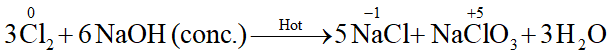Q. Deduce the molecular shape of $B r F_{3}$ on the basis of $V S E P R$ theory.
Ans. - No. of electrons in the valence shell of central $B r$ atom $=7$ No. of electrons provided by three $F$ atoms $=3 \times 1=3$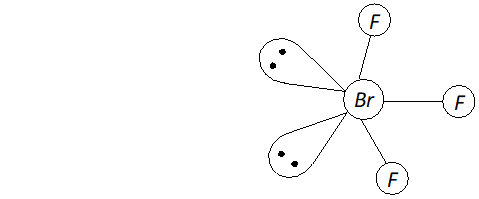Total no. of electrons around the central atom $(B r)=7+3=10$ $\therefore$ Total no. of electron pairs around the central $B r$ atom $=10 / 2=5$ But the no. of bond pairs $=3(\because \text { there are three } B r-F \text { bonds })$ $\therefore$ No. of lone pairs $=5-3=2$ On the basis of $V S E P R$ theory, a molecule with three bond pairs and two lone pairs must be $T$ -shaped (i.e. distorted trigonal bipyramidal) as shown above. The two lone pairs will occupy equatorial positions to minimize lone pair-lone pair and lone pair-bond pair repulsions which are greater than bond pair-bond pair repulsions. Further, the axial $F$ atoms will be slightly bent towards the equatorial $F$ atom to minimize the lone pair-lone pair repulsions. Therefore, the shape of $B r F_{3}$ would be slightly bent $T$ as shown above.
Q. Why are elements of group 18 known as noble gas?
Ans. The elements of group 18 have their valence shell orbitals completely filled. As a result, they react with only a few elements (oxygen and fluorine) only under certain conditions. Therefore, they are called noble gases.
Q. Noble gases have very low boiling points. Why ?
Ans. Noble gases are monoatomic. Their atoms are held together by weak dispersion forces and hence can be liquefied at very low temperatures. Therefore, they have low boiling points.
Q. Does the hydrolysis of $X e F_{6}$ lead to a redox reaction?
Ans. Complete hydrolysis of $\mathrm{XeF}_{6}$ gives $\mathrm{XeO}_{3}$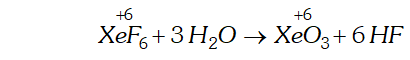On the other hand, partial hydrolysis of $\mathrm{XeF}_{6}$ gives $\mathrm{XeOF}_{4}$ and $\mathrm{XeO}_{2} \mathrm{F}_{2}$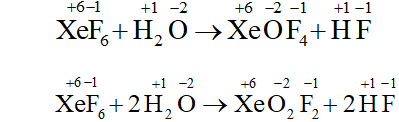Since the oxidation states of all the elements in the products of hydrolysis and reactants is remain the same, therefore, it is not a redox reaction.
Q. Why does the reactivity of nitrogen differ from phosphorus?
Ans. The reactivity of nitrogen is different from phosphorus because of the following reasons : (i) Nitrogen has a small size, high electronegativity, high ionisation enthalpy as compared to phosphorus. (ii) Nitrogen does not contain vacant $d$ -orbitals in its valence shell whereas phosphorus contains vacant $d$ -orbitals in its valence shell. (iii) Nitrogen has ability to form $(N \equiv N)$ triple bond as a result of which its bond enthalpy $\left(941.4 \mathrm{kJmol}^{-1}\right)$ is very high making it less reactive.
Q. Discuss the trends in chemical reactivity of group 15 elements.
Ans. The elements of group 15 differ from one another appreciably in their chemical reactivity. Nitrogen has a very high dissociation energy $\left(941.4 \mathrm{kJmo}^{-1}\right)$ and is practically inert, which is why it has accumulated in large amounts in the atmosphere. Phosphorus, in one of its allotropic forms i.e. white phosphorus is extremely reactive. The strained structure of $P_{4}$ is responsible for high chemical activity. It catches fire when exposed to air forming $P_{4} O_{10} .$ The other allotrope, red phosphorus is stable in air at room temperature, though it reacts on heating. The heavier elements, $A s, S b$ and $B i$ are less reactive. Arsenic is stable in dry air. When heated in air it sublimes at $615^{\circ} \mathrm{C}$ forming $\mathrm{As}_{4} \mathrm{O}_{6}$ Antimony is less reactive and stable towards water and air. On heating in air it forms $S b_{4} O_{6}, S b_{4} O_{8}$ or $S b_{4} O_{10} .$ Bismuth is also known to form $B i_{2} O_{3}$ on heating.
Q. Why does $N H_{3}$ form hydrogen bond but $P H_{3}$ does not?
Ans. In $\mathrm{NH}_{3},$ the nitrogen atoms forms hydrogen bond because of the following reasons. (i) Small size of nitrogen. (ii) High electronegativity of nitrogen ( 3.0) Due to more difference of electronegativity between $N$ and $H$ atom then $N-H$ bond is polar forming $H$ bond. On the contrary, phosphorus due to its larger size and lesser electronegativity ( 2.1) does not form hydrogen bond because $P-H$ bond is almost purely covalent and no hydrogen bond is formed.
Q. How is nitrogen prepared in laboratory? Write chemical equations of the reactions involved.
Ans. Dinitrogen is prepared in laboratory by treating an aqueous solution of ammonium chloride with sodium nitrite. $N H_{4} C l(a q)+N a N O_{2}(a q) \rightarrow N_{2}(g)+2 H_{2} O(l)+N a C l(a q) \quad$ T $\mathrm{h}$ e impurities of $N O$ and $H N O_{3}$ obtained in small amounts is removed by passing the gas through aqueous sulphuric acid containing potassium dichromate.
Q. Illustrate how copper metal can give different products on reaction with $\mathrm{HNO}_{3}$
Ans. The reaction of copper with nitric acid produces different products depending upon the concentration of nitric acid.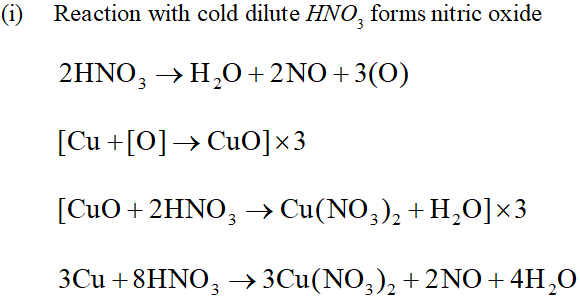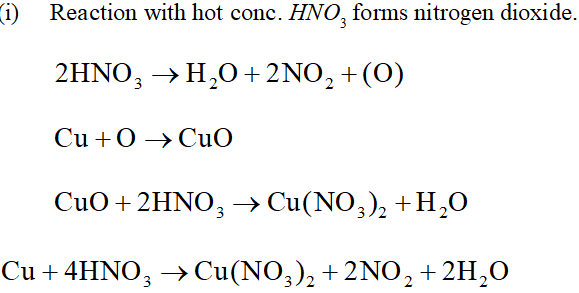Q. Give the resonating structures of $N O_{2}$ and $N_{2} O_{S}$
Ans. The resonating structure of $N O_{2}$ and $N_{2} O_{5}$ are as follows: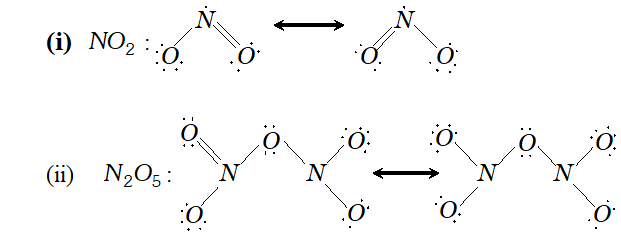Q. The $H N H$ angle value is higher than $H P H, H A s H$ and $H S b H$ angles. Why? [Hint. Can be explained on the basis of $s p^{3}$ hybridisation in $N H_{3}$ and only $s-p$ bonding between hydrogen and other elements of the group]
Ans. The bond angle of the hydrides of group 15 are given below.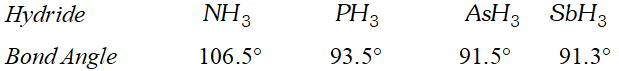The $H N H$ angle in ammonia is higher as compared to other hydrides of the group. It is because nitrogen in is hybridised. However due to lone pair of electrons the bond angle contracts from $109^{\circ} 28^{\prime}$ to $106.5^{\circ} .$ The decreased bond angle in other hydrides is because of the fact that the hybridisation becomes less and less distinct with increasing size of the central atom i.e. pure $p$ -orbitals are utilised in $M-H$ bonding or in simple words the $s$ -orbital of $H$ atom overlaps with orbital having almost pure $p$ -character.
Q. Why does $R_{3} P=O$ exist but $R_{3} N=O$ does not $(R=\text { alky } 1$ group)?
Ans. - Compounds like $R_{3} N=O$ does not exist because of the absence of $d$ -orbitals in the valence shell of nitrogen atom. As a result of this nitrogen cannot expand its covalency beyond 4 (lack of formation of $d \pi-p \pi \text { bond }) .$ On the contrary $R_{3} P=O$ exists because phosphorus has vacant $d$ -orbitals in its valence shell and can expand its covalency beyond 4 by forming bond.
Q. Explain why $N H_{3}$ is basic while $B i H_{3}$ is only feebly basic?
Ans. The hydrides of group 15 are basic in character because of the presence of lone pairs of electrons on the nitrogen atom (Lewis bases $) . N H_{3}$ is strongly basic in character because it can easily donate its electron pair due to small size of nitrogen atom as a result of which electron density of lone pair is concentrated over a small region. $B i H_{3}$ on the contrary is feebly basic because of its larger size. Due to increase in size the electron density gets diffused over a larger region and hence the ability to donate the electron pair decreases.
Q. Nitrogen exists as diatomic molecule and phosphorus as $P_{4}$ why?
Ans. Nitrogen exists as a diatomic molecule $\left(N_{2}\right)$ because due to small size of nitrogen atom and absence of vacant $d$ -orbitals in its valence shell it has strong ability to form multiple bonds $(N \equiv N) .$ The other members of group 15 i.e. phosphorus, Arsenic and antimony all exist as discrete tetraatomic tetrahedral molecules, $v i z, P_{4},$ As $_{4}$ and $S b_{4}$ because these are not capable offorming multiple bonds due to their bigger size and repulsions between non-bonded electrons of the inner core. Now since the angle $P-P-P$ is $60^{\circ},$ the $p \pi-p \pi$ bonding is therefore not possible.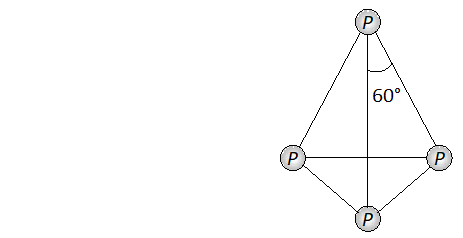Q. Write main differences between the properties of white phosphorus and red phosphorus.
Ans. The difference in properties and structure of white and red phosphorus is given in following table :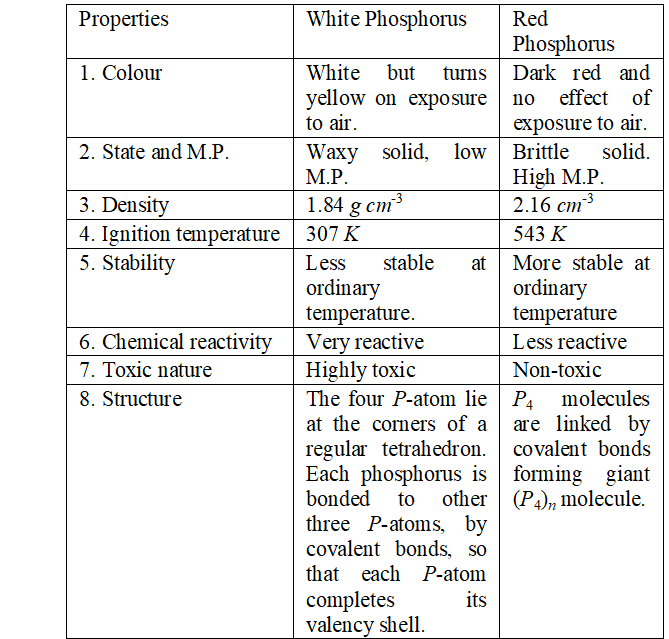Structure of white and red phosphorus are shown below :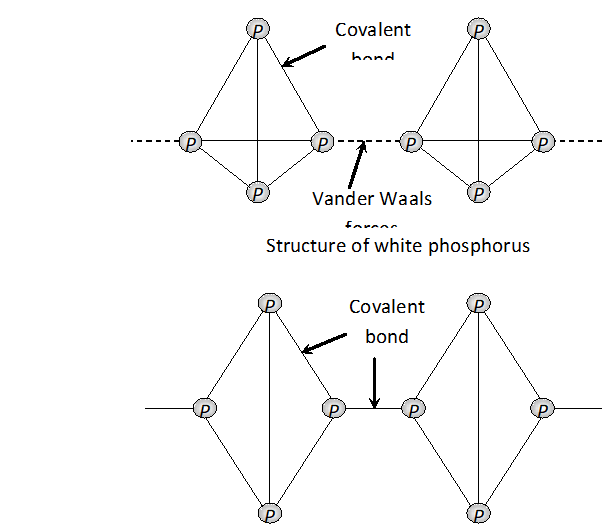Q. Can $P C l_{3}$ act as an oxidising as well as a reducing agent? Justify.
Ans. P C l_{3} can act both as an oxidising agent as well as reducing agent. (a) As a reducing agent. The following reactions support the reducing character $P C l_{3}+S O_{2} C l_{2} \rightarrow P C l_{5}+S O_{2}$ $P C l_{3}+S O_{3} \rightarrow P O C l_{3}+S O_{2}$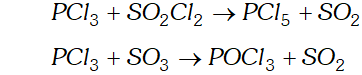(b) As an oxidising agent. It oxidises metals to their respective chlorides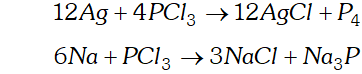Q. Why is dioxygen a gas but sulphur a solid ?
Ans. Dioxygen is a gas because due to small size of oxygen atom and absence of $d$ -orbitals in its valence shell it has strong tendency to form $p \pi-p \pi-p \pi$ bonds multiple bonds $(O=O)$ so as to complete its octet and hence can exist as discrete $\mathrm{O}_{2}$ molecule. On the contrary sulphur is a solid and exists as staggered 8 -atom ring. It is because the tendency of $S=S$ bond formation is missing in sulphur atom due to its larger size and low sulphur-sulphur double bond energy. As a result sulphur atoms complete their octet through formation of $S-S$ bonds in $S_{8}$ molecule. This leads to increase the forces of attraction and hence its physical state is solid.
Q. Knowing the electron gain enthalpy values for $O \rightarrow O^{-}$ and $O \rightarrow O^{2-}$ as $-141$ and $702 k J$ mol $^{-1}$ respectively, how can you account for the formation of a large number of oxides having $Q^{2-}$ species and not $Q^{-} ?$
Ans. The second electron gain enthalpy $\left(O^{-}+e^{-} \rightarrow O^{-2}\right)$ is positive $\left(702 \mathrm{kJ} \mathrm{mol}^{-1}\right)$ yet a large number of oxides have $\mathrm{O}^{2-}$ species. This is attributable to high lattice energy released during formation of such oxides. As a result of this the lattice energy released by the process compensates for the second electron gain enthalpy.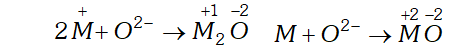Q. Which aerosols deplete ozone?
Ans. The aerosols responsible largely for the depletion of ozone layer are (i) Nitric oxide emitted from the exhaust systems of supersonic jet aeroplanes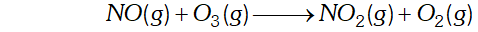Freons (chlorofluoro hydrocarbons) which are used in aerosol sprays and refrigerants.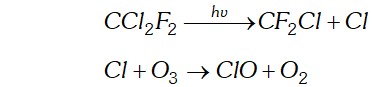Q. How is $S O_{2}$ an air pollutant?
Ans. $-\mathrm{SO}_{2}$ acts as an air pollutant because of the following reasons: (i) is strongly irritating to the respiratory tract. at a concentration of 5 ppm causes throat and eye irritation (resulting into cough, tears and redness in eyes). It causes breathlessness and affects larynx, i.e., voice box. (ii) Even at a low concentration of 0.03 ppm, it has a very damaging effect on the plants. If exposed for a long time, $i . e .,$ a few days or weaks, it slows down the formation of chlorophyll resulting in injury to the leaves including loss of green colour. This is called chlorosis. (iii) dissolves in moisture present in air to form which damages building materials especially marble.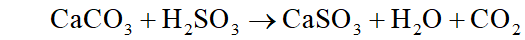It corrodes metals particularly iron and steel. It also brings about fading and deterioration of fabrics, leather, paper, etc. and affecting the colour of paints.
Q. Why are halogens strong oxidising agents ?
Ans. Halogens act as strong oxidising agents because of their high electronegativity values and high electron affinity values. The oxidising power of the halogens is comparable in terms of their reduction potential values given below :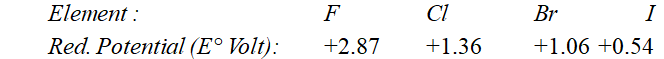As the reduction potential values decrease from fluorine to iodine, the oxidising power also decreases. The reduction potential value depends upon various energy terms as shown below :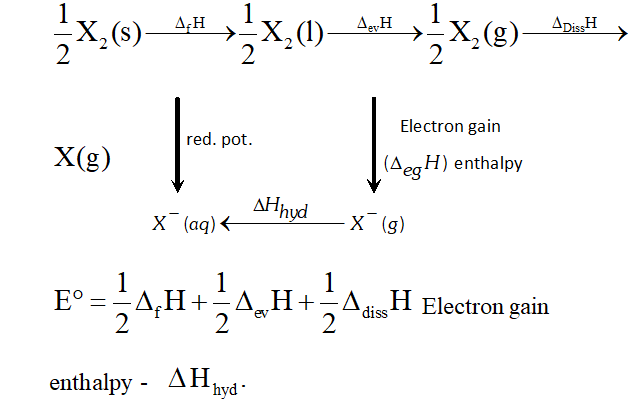Q. Explain why fluorine forms only one oxoacid. HOF.
Ans. Fluorine is known to form only one oxoacid, $H O F$ which is highly unstable. Other halogens form oxoacids of the type $H O X, H X O_{2}$ $H X O_{3},$ and $H X O_{4}(X=C l, B r, I) .$ Fluorine due to its small size and high electronegativity cannot act as central atom in higher oxoacids and hence do not form higher oxoacids.
Q. Write two uses of $\mathrm{ClO}_{2}$
Ans. (i) $\quad \mathrm{ClO}_{2}$ is a powerful oxidising agent and chlorinating agent. Large quantities of are used for bleaching wood pulp and cellulose and for purifying drinking water. (ii) It is an excellent bleaching agent. Its bleaching powder is about 30 times higher than that of and is used for bleaching fluor to make white bread.
Q. Why are halogen coloured ?
Ans. All the halogens are coloured. The colour of halogens deepen with rise in atomic number from fluorine to iodine.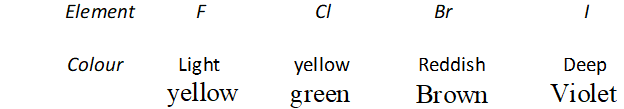The colour is due to absorption of energy from visible light by their molecules for excitation of outer electrons of higher energy levels (the gap of energy between valence shell of halogens and higher energy shells is less). Fluorine absorbs violet portion of the light and appears yellow while iodine absorbs yellow and green portions of the light and thus appears violet. Change in colour on moving from $F$ to $I$ is called blue shift or bathochromic shift.
Q. Write the reactions of $F_{2}$ and $C l_{2}$ with water.
Ans. (i) Fluorine is highly reactive and decomposes water very readily even at low temperature and in dark forming amixture of $O_{2}$ and $O_{3}$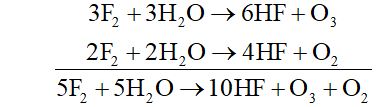(ii) Chlorine decomposes water in the presence of sunlight forming halogen acid and oxoacid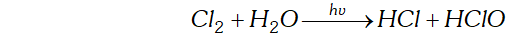Q. What inspired $N .$ Bartlett for carrying out reaction between $X e$ and $P t F_{6} ?$
Ans. N. Bartle $H,$ in $1962,$ prepared a compound by reacting oxygen with $P t F_{6},$ a powerful oxidising agent. The $X$ -ray examination of solid compound, $O_{2} P t F_{6},$ showed that it consisted of $O_{2}^{+}$ and $P t F_{6}^{-}$ ions. Bartle $H$ thought that a similar compound could be prepared with Xenon because the ionisation enthalpy of Xenon is quite close to the ionisation enthalpy of oxygen $\left(1314 \mathrm{kJ} \mathrm{mol}^{-1}\right) .$ Accordingly, he reacted Xenon with $\mathrm{PtF}_{6}$ and got a red solid compound.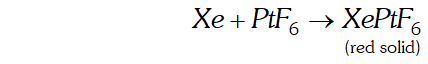Q. What is the oxidation states of phosphorus in the following :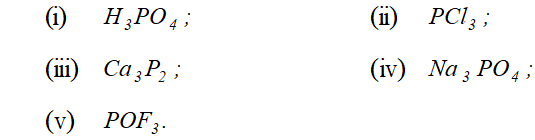Ans. The oxidation state of phosphorus in the compounds is :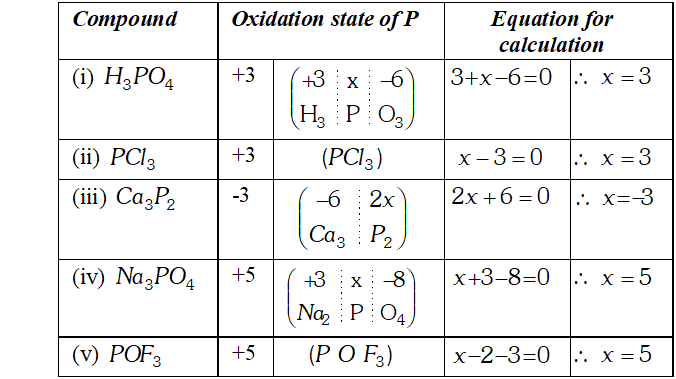Q. How are $\mathrm{XeO}_{3}$ and $\mathrm{XeF}_{4}$ prepared ? Describe their molecular shapes.
Ans. (i) $\quad 6 \mathrm{XeF}_{4}+12 \mathrm{H}_{2} \mathrm{O} \rightarrow 4 \mathrm{Xe}+2 \mathrm{XeO}_{3}+24 \mathrm{HF}+3 \mathrm{O}_{2}$ $$\mathrm{XeF}_{6}+3 \mathrm{H}_{2} \mathrm{O} \rightarrow \mathrm{XeO}_{3}+6 \mathrm{HF}$$ (ii) Partial hydrolysis of $\mathrm{XeF}_{6}$ give $\mathrm{XeOF}_{4}$ $$\mathrm{XeF}_{6}+\mathrm{H}_{2} \mathrm{O} \rightarrow \mathrm{XeOF}_{4}+2 \mathrm{HF}$$ Molecular shape (or structure) $\mathrm{XeO}_{3}$ has a trigonal pyramidal shape due to presence of one lone pair of electrons at one corner of tetrahedron. Similarly, $X e O F_{4}$ has square pyramidal shape. Lone pair of electrons lies opposite to oxygen atom.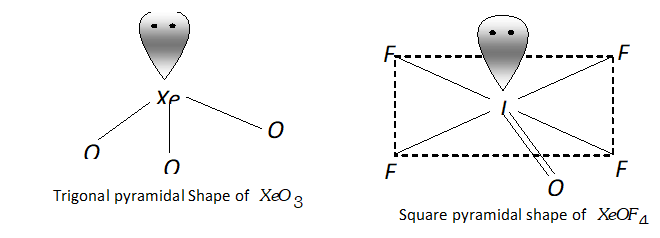Q. Give the formula and describe the structure of a noble gas species which is isostructural with: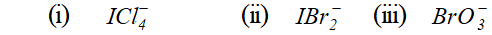Ans. (i) Structure of $I C I_{4}^{-}:$ In $I C I_{4}^{-},$ the central $I$ atom has in all 8 electrons ( 7 valence electrons + one due to negative charge). Four of these form single bonds with four $C l$ atoms (four bond pairs) while the remaining four constitute two lone pairs. Thus, $I$ in has four bond pairs and two lone pairs. Therefore, according to $V S E P R$ theory, it should be square planar as shown.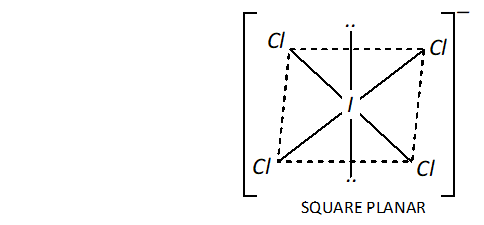Now, $\mathrm{ICl}_{4}^{-}$ has $(7+4 \times 7+1)=36$ valence electrons. A noble gas specieshaving 36 valence electrons in $\mathrm{XeF}_{4}(8+4 \times 7=36)$ Thus, like, is also square planar. (ii) Structure of $I B r_{2}^{-}$ In $, \mathrm{IBr}_{2}^{-}$ the central I atom has 8 electrons (7 valence electrons + one due to negative charge). Two of these form two single bonds (bond pairs) with two $B r$ atoms, while the remaining six constitute three lone pairs. Thus, Iin has two bond pairs and three lone pairs. Therefore, according to $V S E P R$ theory, it should be linear.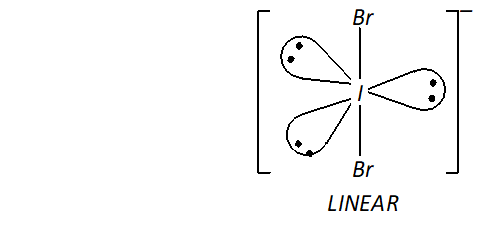Now, $\mathrm{IBr}_{2}^{-}$ has $22(7+2 \times 7+1)$ valence electrons. A noble gas species having 22 valence electrons is $\mathrm{XeF}_{2}(8+2 \times 7=22)$ Thus, like, $\mathrm{IBr}_{2}^{-}, \quad \mathrm{XeF}_{2}$ is also linear. (iii) Structure of $\mathrm{BrO}_{3}^{-}$ The central atom $B r$ has seven electrons. Four of these electrons form two double bonds or coordinate bonds with two oxygen atoms while the fifth electron forms a single bond with The remaining two electrons form one lone pair. Thus, in all there are three bond pairs and one lone pair around Br atom in $\mathrm{BrO}_{3}^{-}$. Therefore, according to $V S E P R$ theory $\mathrm{BrO}_{3}^{-}$ should be pyramidal.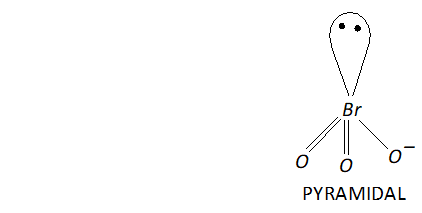Now, $\mathrm{BrO}_{3}^{-}$ has $26(7+3 \times 6+1=26)$ valence electrons. A noble gas species having 26 valence electrons is $\mathrm{XeO}_{3}(8+3 \times 6=26) .$ Thus, like $, \mathrm{BrO}_{3}^{-}, \quad \mathrm{XeO}_{3}$ is also pyramidal.
Q. Why do noble gases have comparatively large atomic sizes?
Ans. The atomic size, in the case of noble gases, is expressed in terms of vander Waal’s radii whereas the atomic size of other members of the period is either metallic radii or covalent radii. As the Vander Waal’s radii is larger than both metallic as well as covalent radii, therefore the atomic size of noble gas is quite large. Among the noble gases, the atomic size increases down the group due to addition of new electronic shells
Q. List the uses of neon and argon gases.
Ans. (i) Uses of neon (a) It is used in neon discharge lamps and signs which are used for advertising purposes. (b) It is used in safety devices for protecting electrical instruments because it has a property of carrying exceedingly high currents under high voltage. (ii) Uses of Argon (a) It is widely used for filling in candescent metal filament electric bulbs. (b) It is used for filling radio-valves, rectifiers and fluorescent tubes. (c) It is used in producing inert atmosphere during welding and extraction of various metals.
Q. Discuss the general characteristics of group 15 elements with reference to their electronic configuration, oxidation state, atomic size, ionisation enthalpy and electronegativity.
Ans. Electronic Configuration : The electronic configurations of the elements of group 15 are given in table. The general valence shell electronic configuration of the elements of group 15 is $n s^{2} n p^{3},$ where $n=2$ to 6 The three electrons in $p$ -orbitals are distributed as $p_{x}^{1}, p_{y}^{1}, p_{z}^{1}$ in accordance with Hund's rule. Electronic configuration of elements of group 15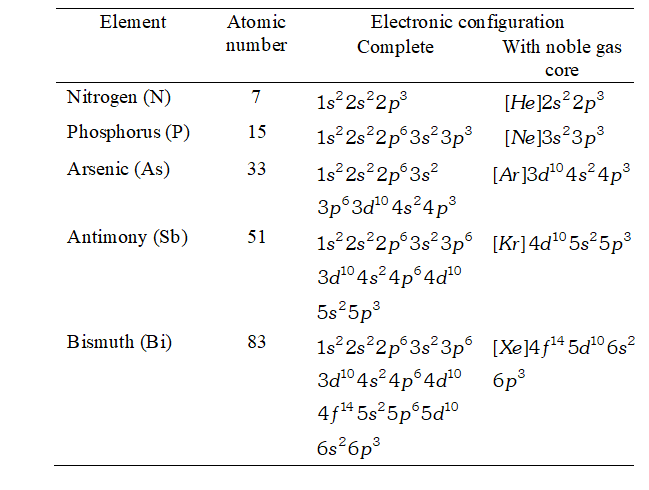(2) Oxidation States (i) Negative oxidation states : All the elements of this group have five electrons in the valence shell $\left(n s^{2} n p^{3}\right)$ and thus require three more electrons to acquire the nearest noble gas configuration. Although gain of three electrons from more metallic elements to form $M^{3-}$ ions requires large amount of energy yet it takes place only with nitrogen because it is the smallest and the most electronegative element of this group. Thus, nitrogen forms $N^{3-(\text { nitride })}$ ion and shows an oxidation state of $-3$ in nitrides of some highly electropositive metals such as $M g_{3} N_{2}, C a_{3} N_{2},$ etc. Other elements of this group form covalent compounds even with metals and show a formal oxidation state of- $-3 .$ For example, calcium phosphide $\left(\mathrm{Ca}_{3} P_{2}\right),$ sodium arsenide $\left(\mathrm{Na}_{3} \mathrm{As}\right),$ zinc stibide $\left(Z n_{3} S b_{2}\right)$ and magnesium bismuthide $\left(M g_{3} B i_{2}\right)$ Besides $-3, N$ and $P$ also show oxidation states of $-2$ in hydrazine $\left(N H_{2} N H_{2}\right)$ and diphosphine $\left(P_{2} H_{4}\right)$ respectively. Nitrogen also shows an oxidation state of $-1$ in hydroxylamine $\left(N H_{2} O H\right)$ but phosphorus does not. (ii) Positive oxidation states : All the elements of this group show positive oxidation states of $+3$ and $+5 .$ However, on moving down the group, the stability of $+5$ oxidation state decreases while that of $+3$ oxidation state increases due to inert pair effect. Thus, $+5$ oxidation state of $B i$ is less stable than $+5$ oxidation state of $S b$ The only stable compound of bismuth having $+5$ oxidation state is $B i F_{s} .$ Further due to large amount of energy needed to lose all the five valence electrons, $M^{5+}$ ions cannot be formed. In other words, all the compounds of group 15 elements having $+5$ oxidation state (i.e. $\left.P F_{5}, P C l_{5}, S b F_{5}, B i F_{5}\right)$ are essentially covalent. However, elements of this group form both ionic ( $i . e . B i F_{3}, S b F_{3}$ ) and covalent compounds (i.e $\left.\mathrm{NC}_{3}, \mathrm{PC}_{3}, \mathrm{AsC}_{3}, \mathrm{SbC}_{3}\right)$ in $+3$ oxidation state. In nut shell, the covalent character decreases in the order: $N>P>A s>S b>B i$ It may, however, be pointed out here that nitrogen because of its small size, high electronegativity and strong tendency to form $p \pi-p \pi-p \pi$ multiple bonds, shows all the oxidation states from $-3$ to $+5$ as shown below: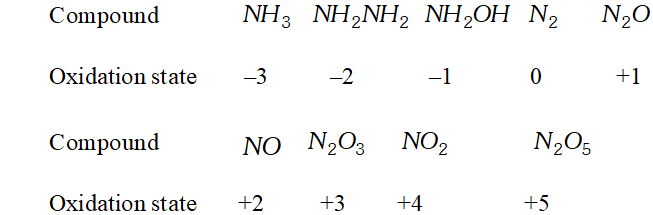(3) Atomic and ionic radii : (i) The atomic (covalent) and ionic radii (in a particular oxidation state) of the elements of nitrogen family (group 15) are smaller than the corresponding elements of carbon family (group 14). Explanation : This is because on moving from left to right, i.e., from group 14 to 15 in a given period, the nuclear charge increases while the new electron enters the same shell. Further, the electrons in the same shell do not screen each other. Therefore, the effective nuclear charge increases and hence the electrons are more strongly attracted towards the nucleus. This results in decrease in covalent radii. Same is true of ionic radii. (ii) On moving down the group, the covalent and ionic radii (in a particular oxidation state) increase with increase in atomic number. There is considerable increase in covalent radius from $N$ to $P .$ However, from $A s$ to $B i,$ only a small increase is observed. Explanation : Down the group, the covalent radii increase primarily due to the addition of a new principal energy shell in each succeeding element. The considerable increase in covalent radius from $N$ to $P$ is not only due to the addition of a new energy shell but is also due to strong shielding effect of the $s-$ and $p$ -electrons present in the inner shells. However, the small increase in covalent radii from $A s$ to $B i$ is due to the poor shielding of the valence electrons by the $d$ -and/or $f$ -electrons present in the inner shells of these heavier elements. As a result, the effective nuclear charge increases which reduces the effect of addition of a new energy shell to some extent. As a result, the increase in covalent radius is small from $A s$ to $B i .$ Same is true of ionic radii. (4) Ionization enthalpy : The ionization enthalpies of the elements of group 15 are much higher than the corresponding elements of group 14 Down the group, the values of the ionization enthalpies decrease regularly. Explanation : Because of increased nuclear charge, reduced atomic radii and stable half-filled electronic configurations, the electrons of group 15 elements are strongly attracted by the nucleus and hence they have less tendency to lose electrons. As a result, ionization enthalpies of the elements of the nitrogen family are much higher as compared to the elements of carbon family. The decrease in their values as we move down the group is due to gradual increase in the atomic size which reduces the force of attraction on the electrons by the nucleus. As expected, successive ionization energies of these elements increase in the order : $\Delta_{i} H_{1}<\Delta_{i} H_{2}<\Delta_{i} H_{3}$ (5) Electronegativity : Group 15 elements are more electronegative than group 14 elements. Electronegativity of elements of group 15 shows a gradual decrease on moving down the group from $N$ to $B i .$ Explanation: As the elements of group 15 have smaller atomic size and need smaller number of electrons to attain noble gas configuration as compared to elements of group $14,$ they are more electronegative. Due to the gradual increase in the atomic size, attraction by the nucleus for the electrons decreases. Hence, their electronegativity values decrease down the group. However, the decrease is not regular.
Q. How is ammonia manufactured industrially ?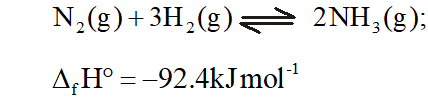Ans. On a commercial scale, ammonia is manufactured by Haber’s process. This reaction is reversible, exothermic and occurs with decrease in volume. Therefore, according to Le Chatelier's principle, the favourable conditions for the manufacture of ammonia are: (i) Low temperature: since the forward reaction is exothermic, low temperature will favour the formation of ammonia. However, at low temperatures, the rat of the reaction will be slow. The optimum temperature for the reaction has been found to be around $700 K$ (ii) High pressure : since the forward reaction occurs with decrease in volume, high pressure will favour the formation of ammonia. The reaction is usually carried out a pressure of about $200 \times 10^{5} \mathrm{Pa}$ or 200 atmospheres. (iii) Catalyst: The rate of reaction is fairly low around $700 \mathrm{K}$. It is increased by using iron oxide as catalyst with small amounts of $K_{2} O$ and $A l_{2} O_{3} .$ Sometimes, molybdenum is used as a promoter (which increases the efficiency of the catalyst). The flow chart for production of ammonia is in figure.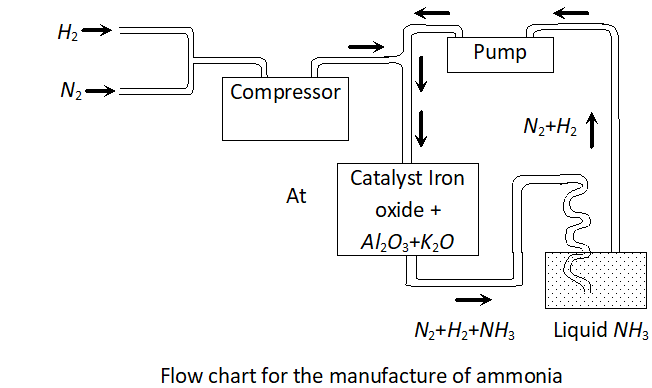Q. Justify the placement of $O . S, S e, T e$ and $P o$ in the same group of the periodic table in terms of electronic configuration, oxidation state and hydride formation.
Ans. Electronic Configuration : The elements of group 16 have six electrons in the valence shell and hence their general outer electronic configuration is $n s^{2} n p^{4}$ The four $p$ -electrons are arranged in three $p$ -orbitals as $p_{x}^{2} p_{y}^{1} p_{z}^{1}$ in accordance with Hund's rule. The names, electronic configurations and common oxidation states of group 16 elements are given in table.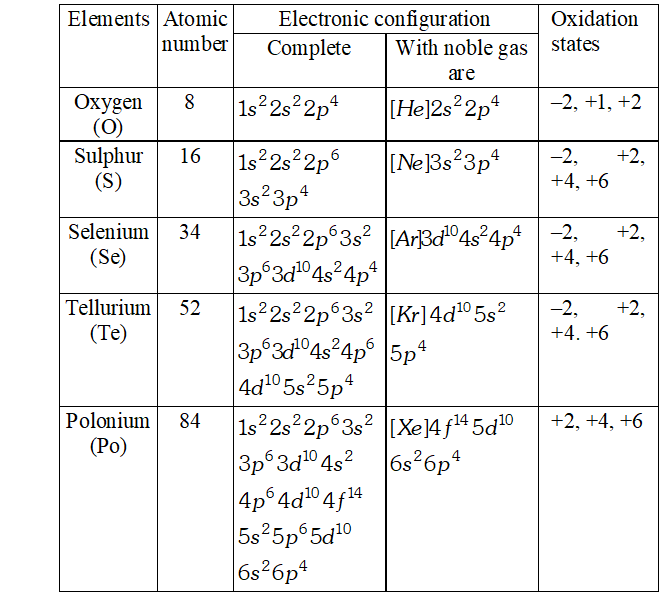(2) Oxidation States : since all the elements of this group have $n s^{2} n p^{4}$ configuration in their valence shell (outer-most shell), they can attain noble gas configuration $v i z . n s^{2} n p^{6}$ either by gaining or by sharing electrons. These elements, therefore, show two types of oxidation states. (i) Negative oxidation states : oxygen the first element of this group, has high electronegativity. Therefore, it preferably completes its octet by gaining of electrons. As a result, all metal oxides are ionic and contain $\mathrm{O}^{2-}$ ions in which oxygen shows an oxidation state of $-2 .$ In addition, oxygen shows an oxidation state of $-1$ in peroxides such as $H_{2} \mathrm{O}_{2}$ zero in $\mathrm{O}_{2}$ and $\mathrm{O}_{3},+1$ in $\mathrm{O}_{2} \mathrm{F}_{2}$ and $+2$ in $O F_{2}$ since the electronegativities decrease as we move down the group, the tendency of these elements to show $-2$ oxidation state decreases from sulphur to polonium. Hence, there is much less probability of the formation of dinegative ions in case of $S,$ Se and $T e$. The least electronegative element, polonium, in fact, does not exhibit negative oxidation state at all. Rather it shows positive oxidation states only. (ii) Positive oxidation states: Oxygen does not show positive oxidation states except in and The other elements of this group show positive oxidation states of $+2,+4$ and $+6$ due to promotion of electrons to vacant $d$ -orbitals as explained in the diagram shown below: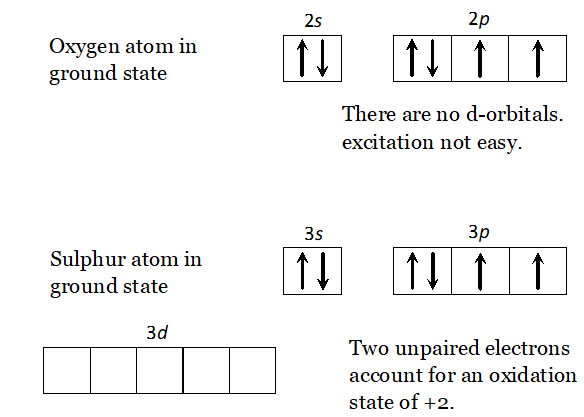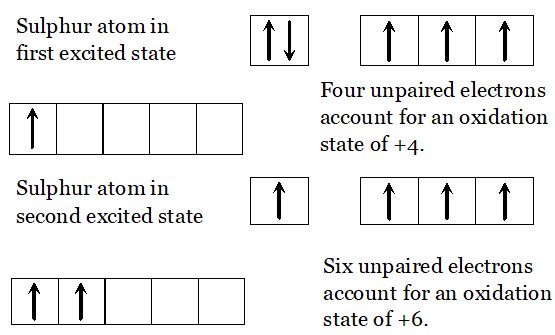In the ground state, these elements have two unpaired electrons and hence can form two bonds. This explains their $+2$ oxidation state. In the first excited state, one of the paired $p$ -electron goes to the vacant $d$ -orbital of the same shell, thus making four unpaired electrons available for chemical bonding. This accounts for their $+4$ oxidation state. On further excitation, one of the s-electrons also gets promoted to $d$ -orbital, thus making six unpaired electrons available for bond formation. This explains their $+6$ oxidation state. However, due to inert pair effect, the stability of $+6$ oxidation state decreases down the group. Thus, $+6$ oxidation state is most stable in case of $S$ and least stable in case of $P O$. In general, the compounds of $S,$ Se, Te and Po with oxygen are tercovalent $(+4 \text { oxidation state). These }+4$ compounds show both oxidising and reducing properties. since fluorine is the strongest oxidising agent, therefore, an element shows its maximum oxidation state in its compound with fluorine. Thus, the compounds of $S,$ Se, Te and Po with fluorine show an oxidation state of $+6$ These compounds in $+6$ oxidations state show only oxidising properties. The behaviour of oxygen is different, since it does not have $d-$ orbitals in its valence shell. Therefore, in case of oxygen, the $2 p-$ electrons on excitation have to go to $3 s$ -orbital. But, since too much energy is required to excite an electron to a higher shell (in this case from $\mathrm{K} \text { to } \mathrm{L}-\text { shell }),$ the electrons in oxygen do not get unpaired. Therefore, oxy gen behaves as a divalent element only. 3. Reactivity towards Hydrogen (Formation of Hydrides). (i) All the elements of group 16 form hydrides of the general formula, $\quad H_{2} E \quad$ where $\quad E=O, S, S e, T e, P o$ $H_{2} \mathrm{O}, \mathrm{H}_{2} \mathrm{S}, \mathrm{H}_{2} \mathrm{Se}, \mathrm{H}_{2} \mathrm{Te}$ and $\mathrm{H}_{2} \mathrm{Po}$ Preparation. The hydrides of $S,$ Se and Te are prepared by the action of acids on metal sulphides, selenides and tellurides respectively. You are already familiar with the Kipp's apparatus used for generation of $H_{2} S$ in the laboratory by the action of dil, $H_{2} \mathrm{SO}_{4}$ on $\mathrm{FeS}$ $F e S(s)+2 H_{3} O^{+}(a q) \rightarrow F e^{2+}(a q)+H_{2} S(g)+2 H_{2} O(l)$ Structure. All these hydrides have angular shape (Fig.) involving $s p^{3}$ -hybridization of the central atom. the bond angles, however, decrease from $H_{2} O$ to $H_{2}$ Te as shown below: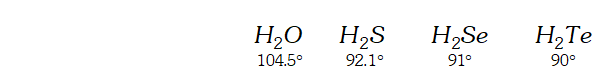Explanation. Due to stronger lone pair-lone pair than bond pairbond pair repulsions, the bond angle in water decreases from the tetrahedral value of $109.28^{\prime}$ to $104.5^{\circ} .$ As we move down the group from $O$ to $T e,$ the size of the central atom goes on increasing and its electronegativity goes on decreasing. As a result, the position of the two bond pairs shifts away and away from the central atom as we move from $H_{2} O$ to $H_{2}$ Te. Consequently the repulsions between the bond pairs decreases as we move from to and, therefore, the bond angle decreases in the same order: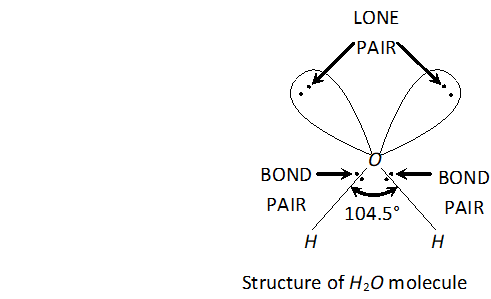$\mathrm{H}_{2} \mathrm{O}>\mathrm{H}_{2} \mathrm{S}>\mathrm{H}_{2} \mathrm{Se}>\mathrm{H}_{2} \mathrm{Te}$ Oxygen forms another important hydride, Some properties of hydrides are given in Table. TABLE : Properties of Hydrides of Group 16 Elements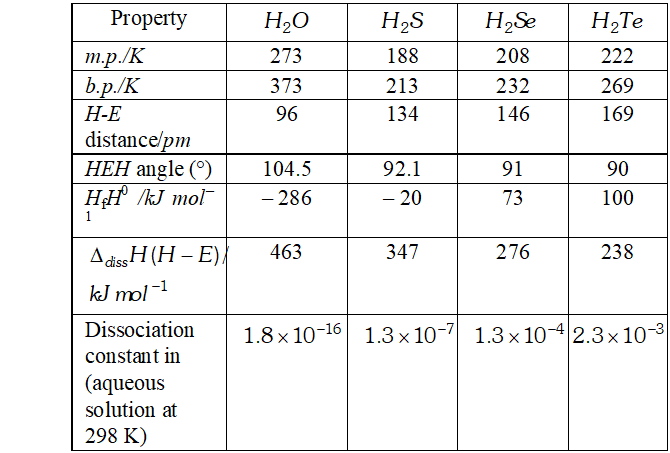Q. Describe the manufacture of $H_{2} S O_{4}$ by contact process?
Ans. $H_{2} S O_{4}$ is mostly prepared by contract process. The acid produced by this process is free from arsenic impurities and is of high purity. The process involves the following steps: (i) Preparation of $\mathrm{SO}_{2}$ by burning of $S$ or roasting pyrites. $S_{8}(s)+8 O_{2}(g) \rightarrow 8 S O_{2}$ $4 \mathrm{FeS}_{2}+11 \mathrm{O}_{2} \rightarrow 2 \mathrm{F}_{2} \mathrm{O}_{3}+8 \mathrm{SO}_{2}$ (ii) Sulphurdioxide gas is purified by passing through the purifying unit. (iii) The pure gas is preheated to $673-723 K$ and passed into catalytic chamber where is oxidised to (iv) gas is then absorbed in conc. in the absorption tower to form oleum which can be diluted with water to get of desired concentration. Favourable conditions for the maximum yield of are: (a) High conc. of oxygen (b) High pressure (c) Low temperature. In about 2 atm. practice, the reaction is carried out at about $625-725 K$ (d) Use of catalyst. To increase the rate of reaction, finely divided platinum or (vanadium pentaoxide) is used as catalyst; (e) Purity of gases. For maximum yield of the most important condition is that reacting gases and must be free from arsenic and other impurities as the impurities act as catalytic poison. Flow diagram of plant is given below :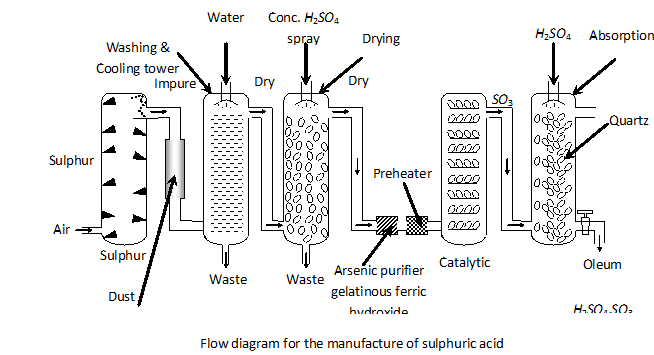Q. Write balanced equations for the following : (i) $\quad$ NaCl is heated with sulphuric acid in the presence of $$M n O_{2}$$ (ii) Chlorine gas is passed into a solution of $N a I$ in water (iii) $\quad \mathrm{SiO}_{2}$ is treated with $H F$ (iv) $\quad \mathrm{NaClO}_{2}$ is treated with $\mathrm{SO}_{2}$ (v) Iodine is treated with conc. $H N O_{3} .$
Ans. (i) Chlorine gas is produced.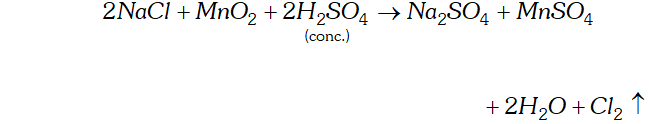(ii) Iodine is liberated. $$2 \mathrm{NaI}+\mathrm{Cl}_{2} \rightarrow 2 \mathrm{NaCl}+\mathrm{I}_{2}$$ (iii) $\quad \mathrm{SiF}_{4}$ is formed. $$\mathrm{SiO}_{2}+4 \mathrm{HF} \rightarrow \mathrm{SiF}_{4}+2 \mathrm{H}_{2} \mathrm{O}$$ (iv) $\quad \mathrm{ClO}_{2}$ gas is produced.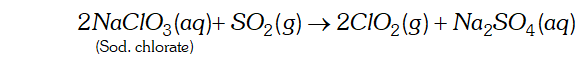(v) $\quad I_{2}$ is oxidised to $H I O_{3}$ $I_{2}+10 H I N O_{3} \rightarrow 2 H I O_{3}+10 N O_{2}+4 H_{2} O$
Q. How are xenon fluorides $X e F_{2}, X e F_{4}$ and $X e F_{6}$ prepared? Deduce their structure applying $V S E P R$ theory.
Ans. Preparation of $\mathrm{XeF}_{2}, \mathrm{XeF}_{4}$ and $\mathrm{XeF}_{6} .$ All three binary fluorides of $X e$ are formed by direct union of elements under approximate experimental conditions. $\mathrm{XeF}_{2}$ can also be prepared by irradiating a mixture of xenon and fluorine with sunlight or light from a pressure-mercury arc lamp.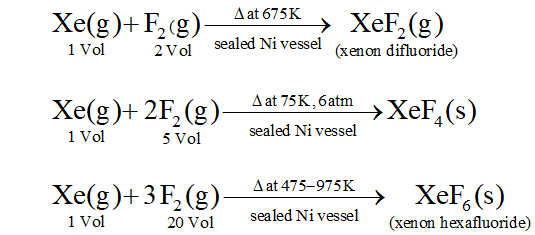Structure of $\mathrm{XeF}_{2} \cdot \mathrm{XeF}_{4}$ and $\mathrm{XeF}_{6}:$ By applying $V S E P R$ theory the shared and unshared pairs of electrons around central atom tends to stay at maximum distance from each other as well as from central atom. On this basis the structures are as follows: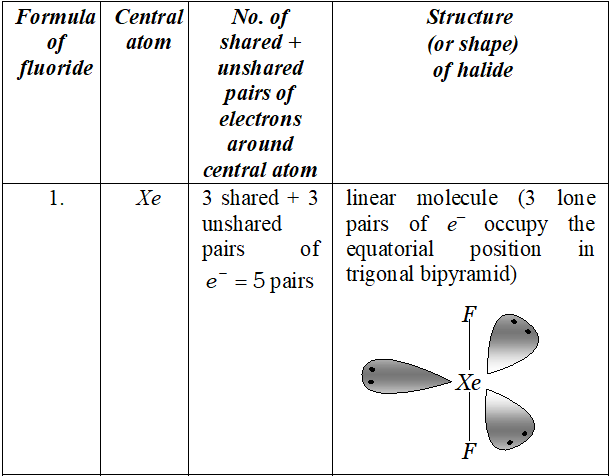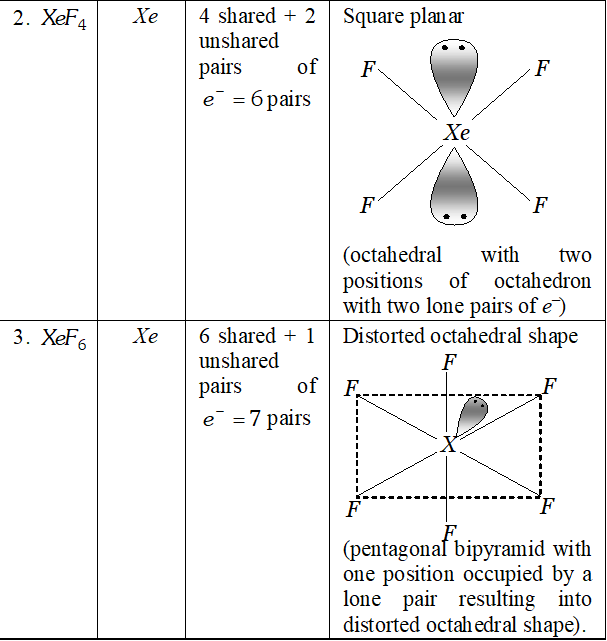Q. Arrange the tollowing in the order of property indicated for each set: (i) $\quad F_{2}, C l_{2}, B r_{2}, I_{2}-$ increasing bond energy. (ii) $\quad H F, H C l, H B r, H I-$ increasing acid strength. (iii) $\quad N H_{3}, P H_{3}, A s H_{3}, S b H_{3}, B i H_{3}-$ increasing base strength (iv) $\quad H_{2} O, H_{2} S, H_{2} S e, H_{2} T e_{3}-$ increasing acid strength
Ans. (i) In the order of increasing bond energy. $\mathrm{Cl}-\mathrm{Cl}$ bond energy is highest while $I-I$ bond energy is least  I-I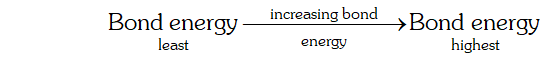(ii) In the order of increasing acid strength in water (i.e., aqueous solution) $H I$ is strongest acid while $H F$ is the weakest acid.